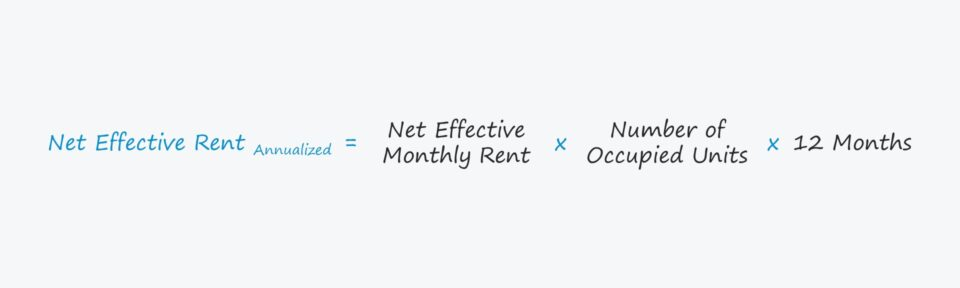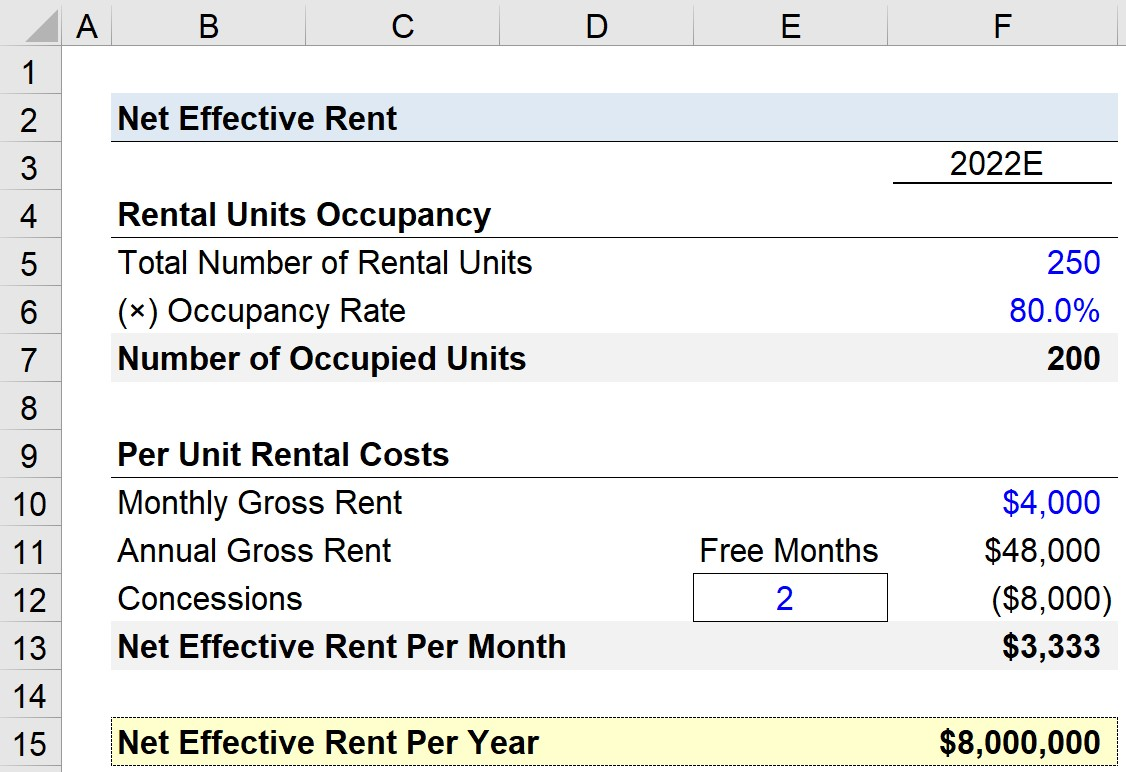# Net Effective Rent

Guide to Understanding the Net Effective Rent## How to Calculate Net Effective Rent

Net effective rent is the amount that a renter pays on a monthly basis for the lease of a rental property such as an apartment unit or rental house.

In order to generate interest from prospective renters and to increase their occupancy rates – i.e. reduce vacancies – landlords often offer concessions or promotions as an added incentive.

While the net effective rent can be presented on a per-month basis, it is standard for real estate owners and investors to annualize the metric as part of their revenue build, which refers to the process of projecting the actual amount of rental income expected to be received from tenants across the term of their leases

Furthermore, most real estate developers and investors own hundreds (or thousands) of units within their portfolios and receive rental payments from all of these tenants.

In a portfolio, the discounts related to concessions and other promotional offers are spread across the entirety of the lease term (and the various tenants).

## Net Effective Rent Formula

The formula for calculating the net effective rent on a monthly basis is as follows.

###### Monthly Net Effective Rent Formula
• Monthly Net Effective Rent = [Gross Rent × (Lease Term – Free Months)] ÷ Lease Term

To reiterate, it is standard for the metric to be annualized for purposes of real estate modeling.

The most practical formula for real estate modeling – in which there is more than one rental unit – is shown below.

###### Net Effective Rent Formula
• Net Effective Rent = Net Effective Rent Per Month × Number of Occupied Units × 12 Months

The annual net effect rent is calculated by taking the monthly net effective rent and multiplying it by the number of units, which is subsequently annualized by multiplying the amount by 12.

## Net Effective Rent vs. Gross Rent

The difference between the net effective rent and the gross rent is that the gross rent – as implied by the name – is the total rent before any adjustments related to concessions or discounts.

When a one-year lease is signed, the gross rent represents the stated rental cost on the rental agreement, either on a monthly or annual basis.

However, the actual rental cost can vary from the stated rental cost because of concessions, discounts, and promotions, which are generally offered during periods when tenant demand in the market is low.

For example, the COVID pandemic led to many rental apartments offering several months free for tenants, as trends such as “work-from-home” were unfavorable to the real estate market (and also as individuals temporarily moved away from cities).

## Net Effective Rent Calculator – Excel Template

We’ll now move to a modeling exercise, which you can access by filling out the form below.Submitting ...

## Net Effective Rent Example Calculation

Suppose an apartment building is projecting its rental income for 2022.

The total number of rental units available for rent is 100 and the anticipated occupancy rate is 85%, so the number of occupied units is 85.

• Total Number of Rental Units = 250
• Occupancy Rate = 80.0%
• Number of Occupied Units = 250 × 80% = 200

Therefore, 200 out of the 250 rental units are occupied and signed to tenants on minimum one-year leases.

The per unit rental costs, i.e. the monthly gross rent – for the sake of simplicity – will be assumed to be priced at \$4,000.

Our next step is to annualize the monthly gross rent by multiplying it by 12 months, which comes out to \$48,000.

• Annual Gross Rent = \$4,000 × 12 Months = \$48,000

If there are no concessions or discounts for any tenants, each tenant would expect to pay \$48,000 in annual rent for 2022. But in our hypothetical scenario, we’ll assume that all tenants in the building were offered two free months (and we’ve created a dropdown list to select between zero and four free months).

The concessions amount to an \$8,000 reduction per unit, which we calculated by multiplying the monthly gross rent by the number of free months.

• Concessions = \$4,000 × 2 Months = \$8,000

The net effective rent, on a monthly basis, is the annual gross rent minus concessions, and then divided by 12.

• Net Effective Rent Per Month = (\$48,000 – \$8,000) ÷ 12 Months = \$3,333

The lease agreement will state the gross monthly rent as \$4,000, yet the actual amount paid by each tenant is \$3,333.

Since we now have all the necessary inputs, we can compute the net effective rent per year by taking the product of the net effective rent per month, the number of occupied units, and the number of months in one year, which enables us to arrive at \$8 million.

The \$8 million in net effective rent per year is the total value of the rental payments expected from the building’s 200 tenants for 2022.

• Net Effective Rent = \$3,333 × 200 Units × 12 Months = \$8,000,00020+ Hours of Online Video Training

#### Master Real Estate Financial Modeling

This program breaks down everything you need to build and interpret real estate finance models. Used at the world's leading real estate private equity firms and academic institutions.